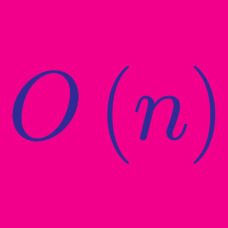Computer Science

# Complexity / Runtime Analysis: Level 3 Challenges

What is the time complexity of the code below?

 1 2 3 4 5 6 int count = 0; for (int i = N; i > 0; i /= 2) { for (int j = 0; j < i; j++) { count += 1; } } 

Assume that arithmetic operations take constant time regardless of the size of the input.

Let $k$ be a fixed constant. You are given a set of $n$ positive integers less than $k$, and you are tasked to sort it.

Which of the following is the asymptotic running time of the fastest possible algorithm?

Ryan is implementing a merge sort algorithm that he heard about in computers class. However, he wasn't paying attention, and ended up implementing the merge sort in a very unusual way.

The standard merge sort takes a list, and recursively splits it in half, until there is only one element left. It then uses the idea that two sorted lists can be easily merged in $O(n)$ time using "two pointer technique" (this step is usually called merge).

Ryan doesn't know about two pointer technique, however, so he decided to replace merge with a bubble sort! The bubble sort runs in $O(n^2)$ time.

What is the runtime of this merge sort implementation?

×

Problem Loading...

Note Loading...

Set Loading...